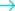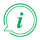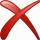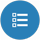Convert volume from Cubic meter to DeciliterConvert from Cubic meter

About Cubic meter to Deciliter converter

Info

To convert from Cubic meter to Deciliter fill in the conversion tool field with the amount you want to convert. The result of Cubic meter to Deciliter conversion will be appeared in the "Result" field in red characters, without need of pressing any button. Below the conversion tool, a list will appear with all the available conversions from Cubic meter.

Examples of Common Queries about converting Cubic meter to Deciliter

Cubic meter to Deciliter converter helps you to find a solution about:
• How do I turn Cubic meter into Deciliter?
• How to convert Cubic meter to Deciliter.
• How to make Cubic meter Deciliter.
• How do I convert Cubic meter volume to Deciliter volume ?
• Is Cubic meter to Deciliter converter free?
• Where can i find Cubic meter to Deciliter converter online.
• Is there a way to convert Cubic meter to Deciliter?